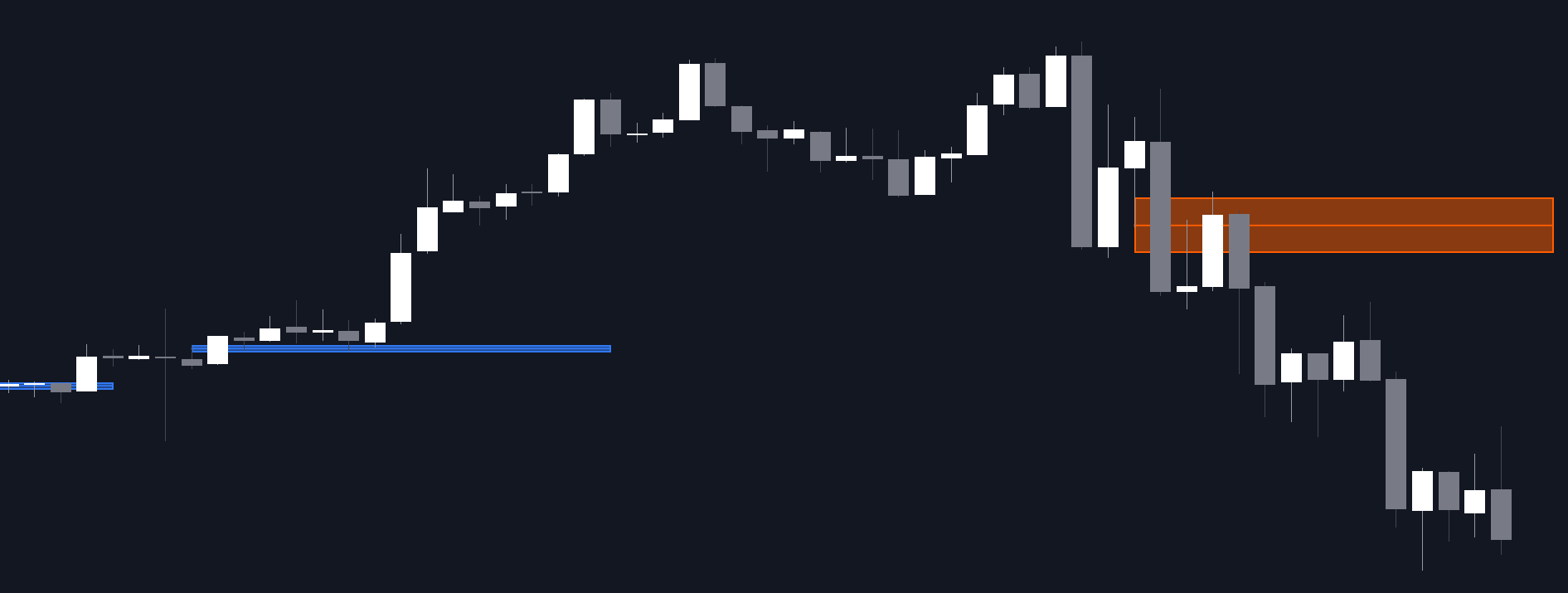# Visible Range Mean Deviation Histogram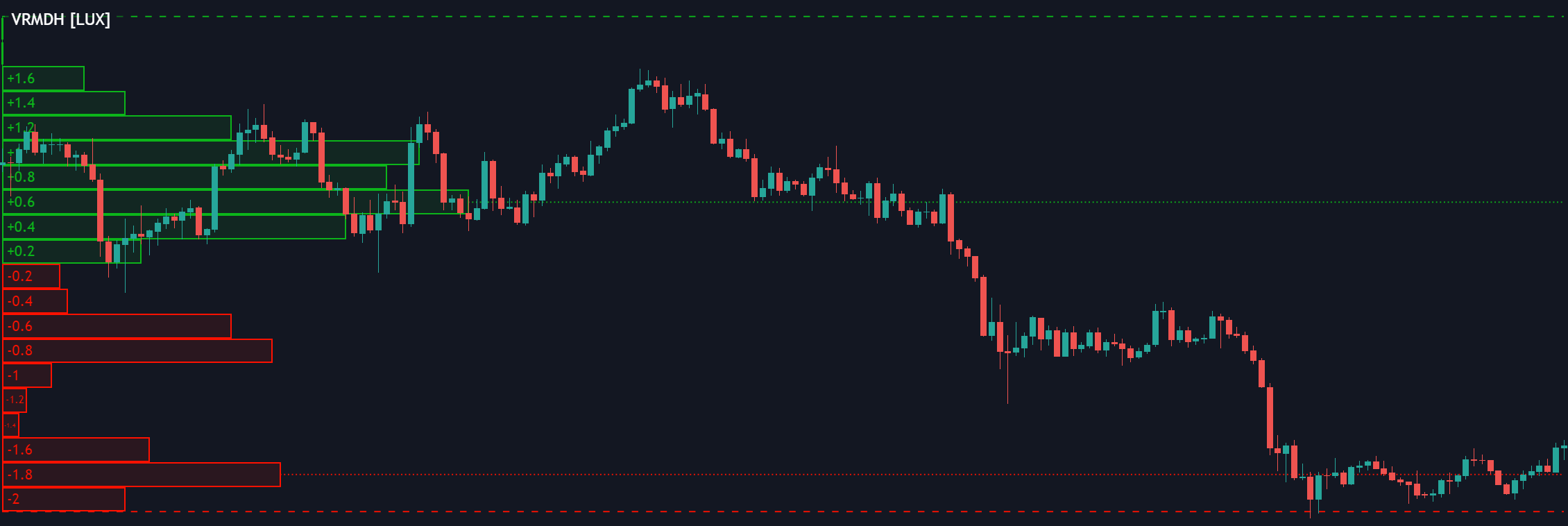This script displays a histogram from the mean and standard deviation of the visible price values on the chart. Bin counting is done relative to high/low prices instead of counting the price values within each bin, returning a smoother histogram as a result.

This script displays a histogram from the mean and standard deviation of the visible price values on the chart. Bin counting is done relative to high/low prices instead of counting the price values within each bin, returning a smoother histogram as a result.

#### Settings

• Bins Per Side: Number of bins computed above and below the price mean
• Deviation Multiplier: Standard deviation multiplier
##### Style
• Relative: Determines whether the bins length is relative to the maximum bin count, with a length controlled with the width settings to the left.
• Bin Colors: Bin/POC Lines colors
• Show POCs: Shows point of controls

#### Usage

Histograms are generally used to estimate the underlying distribution of a series of observations, their construction is generally done taking into account the overall price range.

The proposed histogram construct N intervals above*below the mean of the visible price, with each interval having a size of: σ × Mult / N, where σ is the standard deviation and N the number of Bins per side and is determined by the user. The standard deviation multipliers are highlighted at the left side of each bin.

A high bin count reflects a higher series of observations laying within that specific interval, this can be useful to highlight ranging price areas.

POCs highlight the most significant bins and can be used as potential support/resistances.

## Recent posts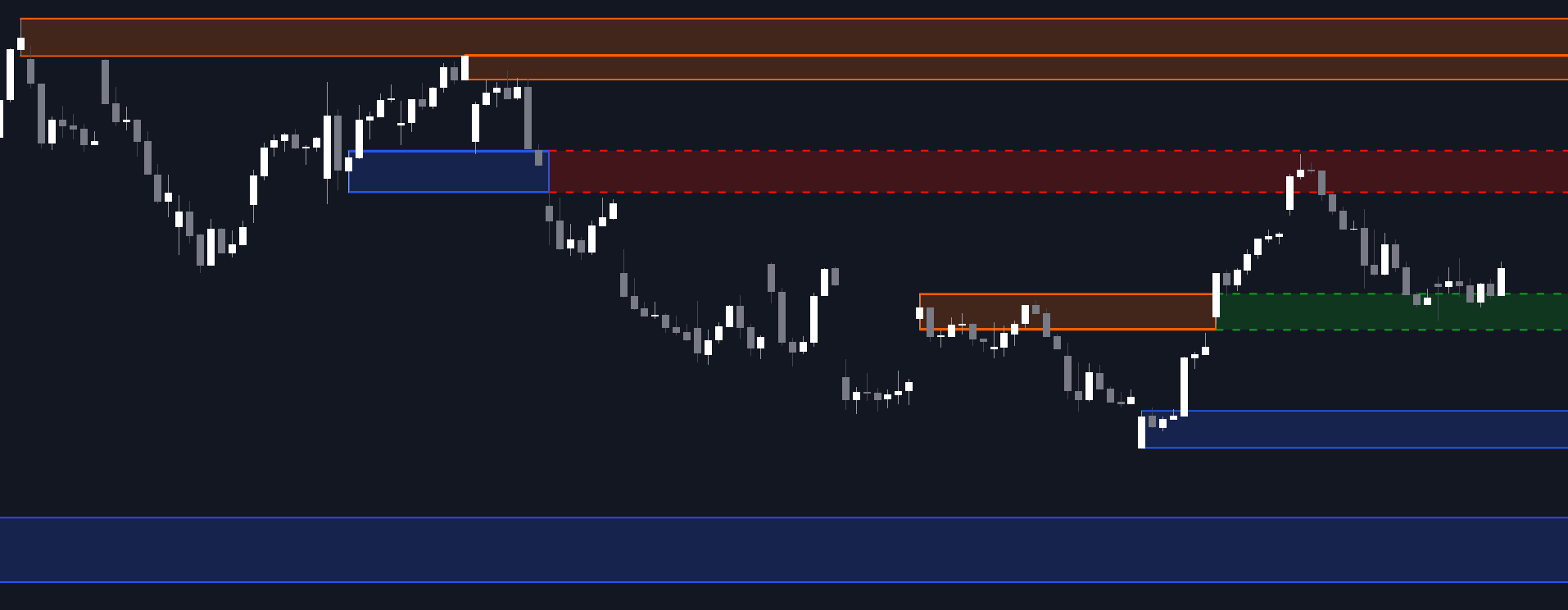### Order Blocks & Breaker Blocks

##### March 9, 2023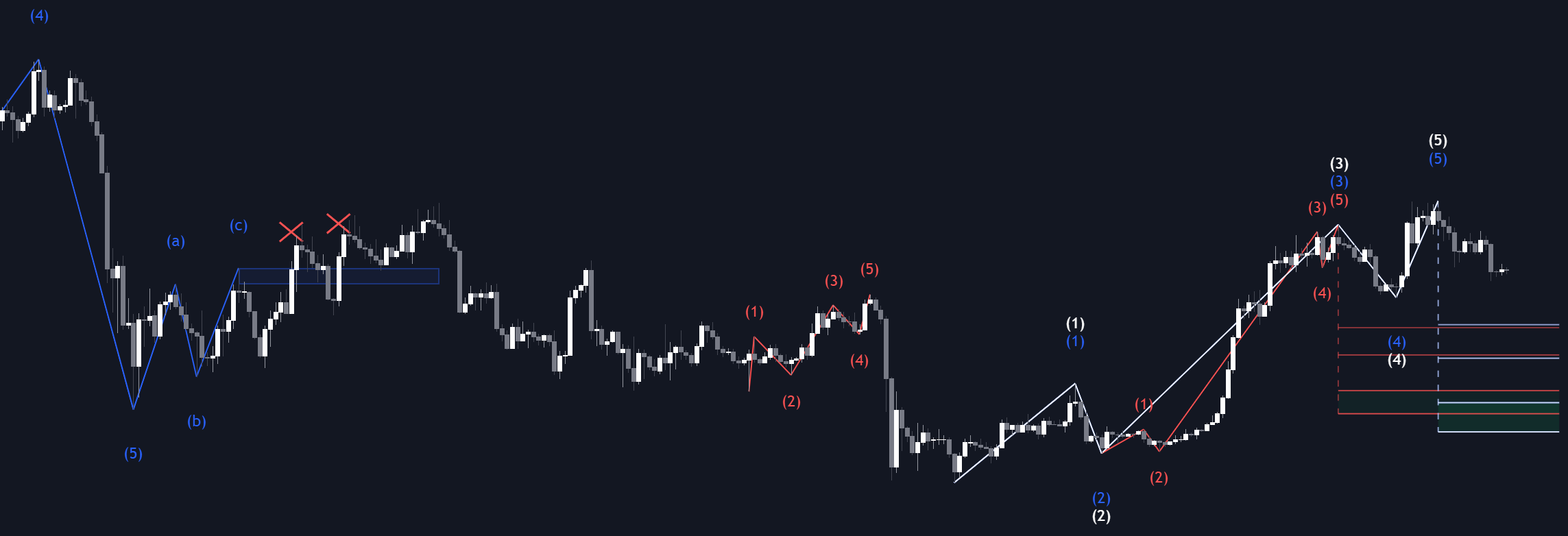### Elliott Wave

##### March 3, 2023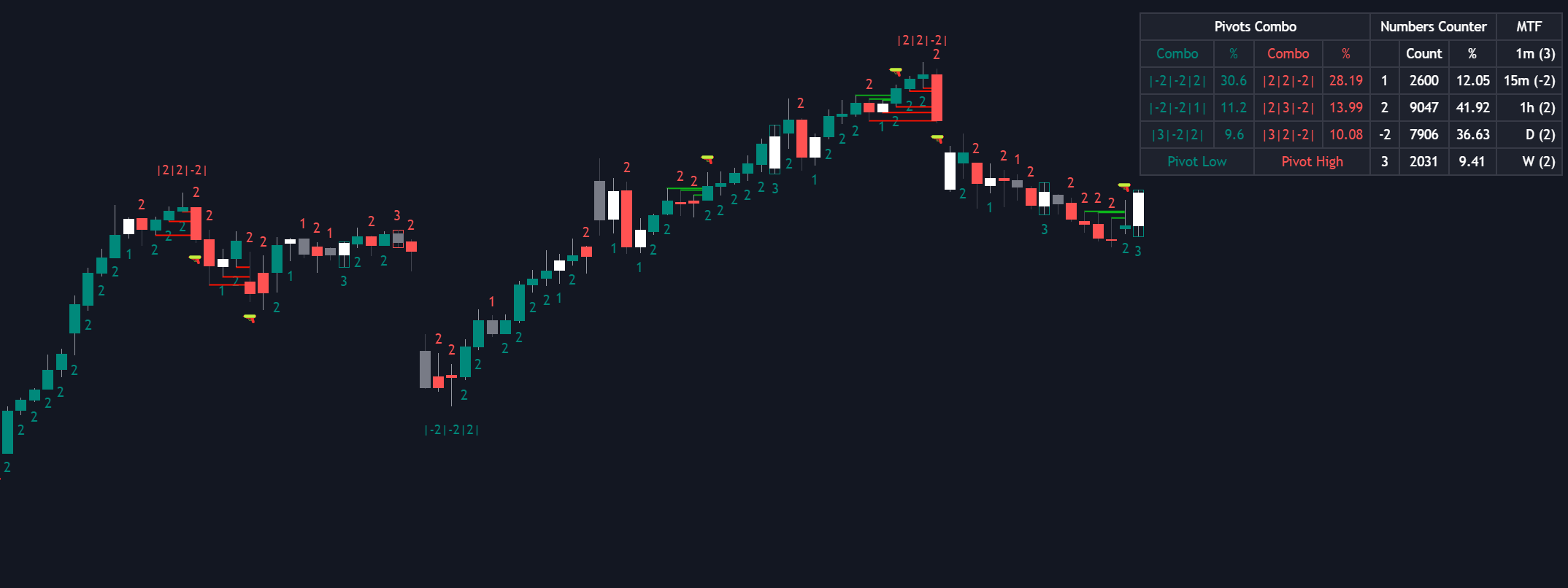### The Strat

##### March 2, 2023